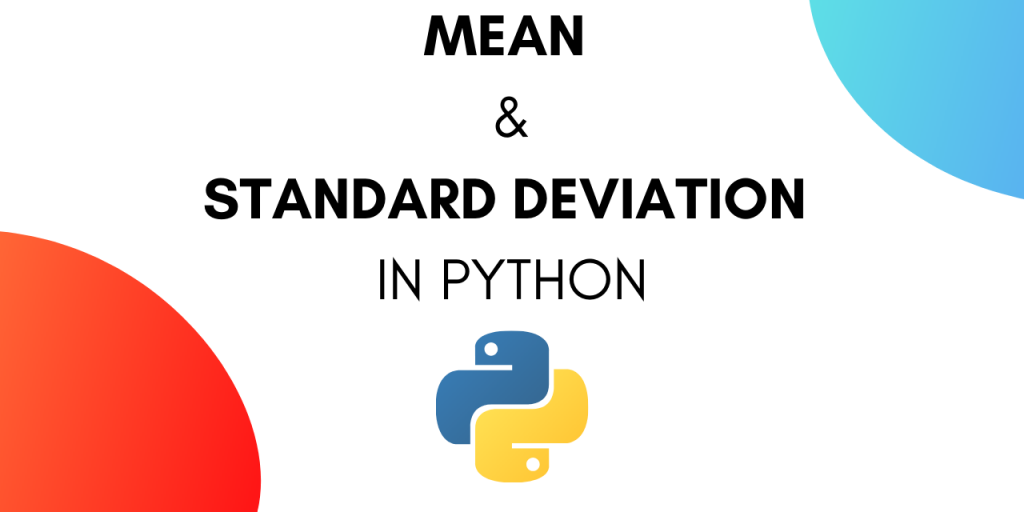# Mean and Standard Deviation in PythonMean and standard deviation are two essential metrics in Statistics. We can use the statistics module to find out the mean and standard deviation in Python. Standard deviation is also abbreviated as SD.

## What is Mean?

The mean is the sum of all the entries divided by the number of entries. For example, if we have a list of 5 numbers [1,2,3,4,5], then the mean will be (1+2+3+4+5)/5 = 3.

## What is Standard Deviation?

Standard deviation is a measure of the amount of variation or dispersion of a set of values. We first need to calculate the mean of the values, then calculate the variance, and finally the standard deviation.

## Uses of Standard Deviation

Let’s say we have the data of population per square kilometer for different states in the USA. We can calculate the standard deviation to find out how the population is evenly distributed. A smaller value means that the distribution is even whereas a larger value means there are very few people living in some places while some areas are densely populated.

Let’s look at the steps required in calculating the mean and standard deviation.

## Steps to Calculate Mean

1. Take the sum of all the entries.
2. Divide the sum by the number of entries.

## Steps to Calculate Standard Deviation

1. Calculate the mean as discussed above. The mean of [1, 2, 3, 4, 5] is 3.
2. Calculate variance for each entry by subtracting the mean from the value of the entry. So variance will be [-2, -1, 0, 1, 2].
3. Then square each of those resulting values and sum the results. For the above example, it will become 4+1+0+1+4=10.
4. Then divide the result by the number of data points minus one. This will give the variance. So variance will be 10/(5-1) = 2.5
5. The square root of the variance (calculated above) is the standard deviation. So standard deviation will be sqrt(2.5) = 1.5811388300841898.

## Find the Mean and Standard Deviation in Python

Let’s write the code to calculate the mean and standard deviation in Python. We will use the statistics module and later on try to write our own implementation.

### 1. Using the statistics module

This module provides you the option of calculating mean and standard deviation directly.

Let’s start by importing the module.

```import statistics
```

Let’s declare a list with sample data.

```data = [7,5,4,9,12,45]
```

Now to calculate the mean of the sample data, use the following function:

```statistics.mean(data)
```

This statement will return the mean of the data. We can print the mean in the output using:

```print("Mean of the sample is % s " %(statistics.mean(data)))

```

We get the output as:

```Mean of the sample is 13.666666666666666
```

If you are using an IDE for coding you can hover over the statement and get more information on statistics.mean() function.

Alternatively, you can read the documentation here.

To calculate the standard deviation of the sample data use:

```print("Standard Deviation of the sample is % s "%(statistics.stdev(data)))
```

We get the output as:

```Standard Deviation of the sample is 15.61623087261029
```

Here’s a brief documentation of statistics.stdev() function.

### Complete Code to Find Standard Deviation and Mean in Python

The complete code for the snippets above is as follows :

```import statistics

data = [7,5,4,9,12,45]

print("Standard Deviation of the sample is % s "% (statistics.stdev(data)))
print("Mean of the sample is % s " % (statistics.mean(data)))
```

### 2. Write Custom Function to Calculate Standard Deviation

Let’s write our function to calculate the mean and standard deviation in Python.

```def mean(data):
n = len(data)
mean = sum(data) / n
return mean
```

This function will calculate the mean.

Now let’s write a function to calculate the standard deviation.

This can be a little tricky so let’s go about it step by step.

The standard deviation is the square root of variance. So we can write two functions:

• the first function will calculate the variance
• the second function will calculate the square root of the variance and return the standard deviation.

The function for calculating variance is as follows:

```def variance(data):

n = len(data)

mean = sum(data) / n

deviations = [(x - mean) ** 2 for x in data]

variance = sum(deviations) / n
return variance
```

You can refer to the steps given at the beginning of the tutorial to understand the code.

Now we can write a function that calculates the square root of variance.

```def stdev(data):
import math
var = variance(data)
std_dev = math.sqrt(var)
return std_dev
```

### Complete Code

The complete code is as follows :

```import numpy as np #for declaring an array or simply use list

def mean(data):
n = len(data)
mean = sum(data) / n
return mean

def variance(data):
n = len(data)
mean = sum(data) / n
deviations = [(x - mean) ** 2 for x in data]
variance = sum(deviations) / n
return variance

def stdev(data):
import math
var = variance(data)
std_dev = math.sqrt(var)
return std_dev

data = np.array([7,5,4,9,12,45])

print("Standard Deviation of the sample is % s "% (stdev(data)))
print("Mean of the sample is % s " % (mean(data)))
```

## Conclusion

The mean and Standard deviation are mathematical values used in statistical analysis. Python statistics module provides useful functions to calculate these values easily.